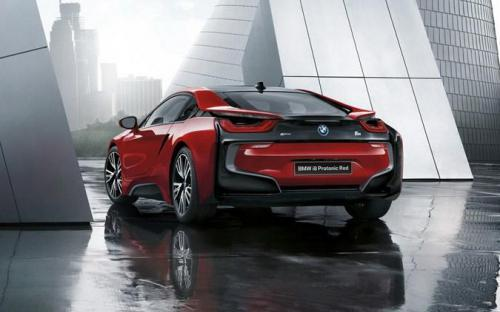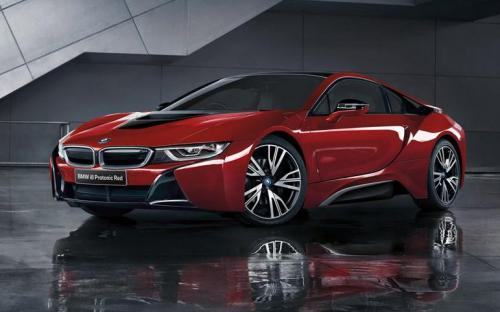### 宝马(进口) 宝马i82016款最低售价：209.80 万元起

4689(mm)1942(mm)1299(mm)##### 配置亮点：
• 胎压监测装置

• ISOFIX儿童座椅接口

• 车身稳定控制(ESC/ESP/DSC等)

• 电动天窗

• 定速巡航

• 后倒车雷达

• 真皮座椅

• GPS导航系统

• 氙气大灯

• 后视镜加热

• 提交
2016款 质子红限量版 (67张)

宝马(进口) 宝马i8 绕车实拍• 宝马(进口) 宝马i8 在售车型

排量 车型 厂商指导价 本地最低报价 购车工具
T
质子红限量版 插电式混合动力 6挡手自一体
209.80万
1.5T
质子红限量版 插电式混合动力 6挡手自一体
209.80万

宝马(进口) 宝马i8 经销商

查看更多 >>

### 宝马(进口) 宝马i8 动力加速

宝马i8 0-100公里加速时间分布在 4.4-4.4秒 属于 高性能级

动力级别 加速时间 车型
高性能级(1款)
4.4s
质子红限量版标准型

宝马(进口) 宝马i8 视频

宝马(进口) 宝马i8 新闻资讯

# 懂车帝真人车友当主播 美女车友试驾宝马i8

评测 超过11535次关注

12月7日，懂车帝邀请车友圈美女车友“小珍珠”进行真人试驾宝马i8直播，通过“小珍珠”的体验与观看，让受众了解到i8的真实情况，超过15万车友在线收看了直播。

# 宝马研发i系列车型 2020年或推出第三款

进口新车 超过7321次关注

宝马研发方面的负责人KlausFroehlich在接受采访时表示，宝马目前已经着手第三款i系列车型的研发工作，该车将会在2020年左右与消费者见面。目前，该车的研发仍处于比...

# 颠覆传统的跑车 抢先试驾2014款宝马i8

评测 超过6894次关注

就在近日，我们从上海上德宝骏宝马5S店获悉，这台混合动力跑车已然到店，并已静静地停放在了i系列展示区中。而此前，我的同事苑璐也有幸简单试驾了宝马i8，结合在一...

# 埃森改装展：宝马i8改装轮圈和减振套件

改装 超过3067次关注

德国埃森改装展正在进行中，宝马改装专家Manhart的展台上展出了一辆宝马i8改装案例，主要改装了外观、操控和轮圈。

# 主流新能源车企纷纷抢登陆

新闻中心 超过4491次关注

2014年，可谓中国新能源汽车高速发展的一年，一系列利好推动新能源市场的繁荣。

# 采用对开门设计 宝马i8轿车版假想图

新闻 超过7058次关注

近日，有海外媒体绘制了一副宝马i8轿车版车型的假想图，新车采用了和宝马i3车型相似的对开门设计。

# 45万/200万起 宝马i3/i8将9月21日上市

新闻 超过4907次关注

日前，我们获得消息宝马i3和i8将会于9月21日正式上市，其中i3的预计起售价为45万，i8的预计起售价为200万元左右。目前宝马已经在北京、上海、深圳和沈阳正式授权7家...

# 0-100km/h加速3.5秒 宝马2017年将推i8S

新闻 超过8083次关注

宝马i8已于9月份在国内正式上市，售价为198.8万元。日前，海外媒体报道称宝马公司正在基于这款车开发高性能版本车型，这款车或被命名为i8S，将会在2017年发布。

# 宝马i8改装方案设计图

玩车用车 超过8537次关注

德国改装公司GermanSpecialCustoms（简称GSC）近日推出了宝马i8改装案例的效果图，从公布的信息中看到，GSC为这辆车设计了一套更加激进的外观套件。

猜你喜欢

﻿
• 快速找车
• 选择品牌
• 选择品牌
• A  奥迪
• A  阿斯顿·马丁
• A  阿尔法·罗密欧
• B  宝沃
• B  布加迪
• B  巴博斯
• B  保时捷
• B  宾利
• B  奔驰
• B  宝马
• B  本田
• B  别克
• B  标致
• B  比亚迪
• B  宝骏
• B  北汽制造
• B  北汽新能源
• B  北汽幻速
• B  北汽威旺
• B  北京汽车
• B  奔腾
• B  北汽绅宝
• C  长安
• C  长安商用
• C  长城
• C  昌河
• D  大众
• D  道奇
• D  DS
• D  东南
• D  东风风神
• D  东风风行
• D  东风小康
• D  东风风度
• D  东风
• F  福特
• F  丰田
• F  菲亚特
• F  法拉利
• F  福田
• F  福迪
• F  福汽启腾
• G  观致
• G  广汽传祺
• G  广汽吉奥
• G  GMC
• H  红旗
• H  汉腾汽车
• H  哈弗
• H  哈飞
• H  海格
• H  海马
• H  华颂
• H  黄海
• H  华泰
• H  恒天
• J  吉利汽车
• J  捷豹
• J  Jeep
• J  江淮
• J  江铃
• J  金杯
• J  九龙
• J  金旅
• K  凯翼
• K  凯迪拉克
• K  克莱斯勒
• K  科尼塞克
• K  卡威
• K  开瑞
• L  路虎
• L  林肯
• L  劳斯莱斯
• L  兰博基尼
• L  雷克萨斯
• L  铃木
• L  雷诺
• L  理念
• L  力帆
• L  莲花汽车
• L  猎豹
• L  路特斯
• L  陆风
• M  马自达
• M  MG
• M  MINI
• M  玛莎拉蒂
• M  摩根
• M  迈凯轮
• N  纳智捷
• O  欧宝
• O  讴歌
• O  欧朗
• Q  奇瑞
• Q  起亚
• Q  启辰
• R  日产
• R  荣威
• R  瑞麒
• S  三菱
• S  斯威汽车
• S  萨博
• S  smart
• S  斯柯达
• S  斯巴鲁
• S  思铭
• S  双龙
• S  上汽大通
• S  双环
• T  特斯拉
• T  腾势
• W  沃尔沃
• W  五菱汽车
• W  五十铃
• W  威兹曼
• W  威麟
• X  现代
• X  雪佛兰
• X  雪铁龙
• X  西雅特
• Y  一汽
• Y  英菲尼迪
• Y  英致
• Y  依维柯
• Y  野马汽车
• Y  永源
• Z  众泰
• Z  中华
• Z  中兴
• Z  知豆
• 选择车系
• 选择车系
• 车型对比
• 选择品牌
• 选择品牌
• A  奥迪
• A  阿斯顿·马丁
• A  阿尔法·罗密欧
• B  宝沃
• B  布加迪
• B  巴博斯
• B  保时捷
• B  宾利
• B  奔驰
• B  宝马
• B  本田
• B  别克
• B  标致
• B  比亚迪
• B  宝骏
• B  北汽制造
• B  北汽新能源
• B  北汽幻速
• B  北汽威旺
• B  北京汽车
• B  奔腾
• B  北汽绅宝
• C  长安
• C  长安商用
• C  长城
• C  昌河
• D  大众
• D  道奇
• D  DS
• D  东南
• D  东风风神
• D  东风风行
• D  东风小康
• D  东风风度
• D  东风
• F  福特
• F  丰田
• F  菲亚特
• F  法拉利
• F  福田
• F  福迪
• F  福汽启腾
• G  观致
• G  广汽传祺
• G  广汽吉奥
• G  GMC
• H  红旗
• H  汉腾汽车
• H  哈弗
• H  哈飞
• H  海格
• H  海马
• H  华颂
• H  黄海
• H  华泰
• H  恒天
• J  吉利汽车
• J  捷豹
• J  Jeep
• J  江淮
• J  江铃
• J  金杯
• J  九龙
• J  金旅
• K  凯翼
• K  凯迪拉克
• K  克莱斯勒
• K  科尼塞克
• K  卡威
• K  开瑞
• L  路虎
• L  林肯
• L  劳斯莱斯
• L  兰博基尼
• L  雷克萨斯
• L  铃木
• L  雷诺
• L  理念
• L  力帆
• L  莲花汽车
• L  猎豹
• L  路特斯
• L  陆风
• M  马自达
• M  MG
• M  MINI
• M  玛莎拉蒂
• M  摩根
• M  迈凯轮
• N  纳智捷
• O  欧宝
• O  讴歌
• O  欧朗
• Q  奇瑞
• Q  起亚
• Q  启辰
• R  日产
• R  荣威
• R  瑞麒
• S  三菱
• S  斯威汽车
• S  萨博
• S  smart
• S  斯柯达
• S  斯巴鲁
• S  思铭
• S  双龙
• S  上汽大通
• S  双环
• T  特斯拉
• T  腾势
• W  沃尔沃
• W  五菱汽车
• W  五十铃
• W  威兹曼
• W  威麟
• X  现代
• X  雪佛兰
• X  雪铁龙
• X  西雅特
• Y  一汽
• Y  英菲尼迪
• Y  英致
• Y  依维柯
• Y  野马汽车
• Y  永源
• Z  众泰
• Z  中华
• Z  中兴
• Z  知豆
• 选择车系
• 选择车系
• 选择车型
• 选择车型
• 意见反馈